Study Guide

# Rational Functions and Their Graphs

## Rational Functions and Their Graphs

Did we mention rational functions and their graphs yet? Naw, we wanted to ease you into this new realm of knowledge. But what, pray tell, does this new lingo mean?### What the Heck Are Rational Functions?

Just a quick reminder about what we've been talking about. Remember these guys?

A rational function is defined as: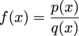...where p(x) and q(x) are different polynomial functions and q(x) ≠ 0.

• ### Evaluating Rational Functions

It doesn't take a lot of heavy mathematical lifting to work through rational functions. We just plug in numbers. That's it—at this stage of the game, anyway. Once we figure out how to calculate the solution of a rational function given a value, we'll become graph-making machines.

### Sample Problem

If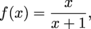find f(0), f(1), and f(2).

To find f(0), which by the way is read "f of 0," we plug 0 in for all the x's in the function.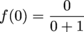Which means:

f(0) = 0

Next up, we plug in x = 1 to find f(1).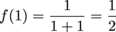Finally, we track down f(2).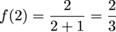### Sample Problem

If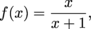find f(-3), f(-2), and f(-1).

If you're in the mood to go negative, you can, and you can have fun with it. Here's how to evaluate rational functions for negative numbers.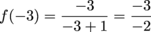Which means: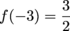How 'bout f(-2)?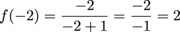Yep, that's how it's done. Lastly, we find f(-1).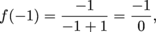...which means it's undefined at that value. Dividing by 0 is the ultimate no-no.

### Sample Problem

If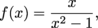find f(-2), f(-1), and f(2).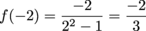Onward to f(-1), which tells us to plug in x = -1.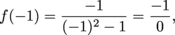...which means it's undefined. Boo. And finally, f(2) gets a chance to shine.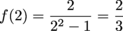• ### Graphing Rational Functions

Don't worry if the last time you graphed something was when you were tracking the life and hard times of your ever-shrinking bank account. Graphing rational functions is not rocket science, and it won't break the bank (unlike that last purchase—a sweet ukulele).When we evaluate a function for specific values, we're finding an ordered pair (think Tweedle Dee and Tweedle Dum, Mary Kate and Ashley, peanut butter and jelly). If we find that f(0) = 2, for example, that means the point (0, 2) is on our graph.

If we get a big, fat "undefined" for a specific value of x, that shows us where the graph's asymptotes are. Asym—what?

To translate from math-speak, an asymptote is an imaginary line that a graph approaches but never touches. The graph will just get closer and closer to the asymptote. Close, but no banana.

Vertical asymptotes happen wherever the denominator is zero, which makes the fraction undefined. (Remember that no-no?) We draw vertical asymptotes as dotted vertical lines, and our curve shouldn't ever quite hit those dotted lines.

Intercepts also help us in our graphing endeavors. We find the y-intercept of the function by solving for f(0), which tells us where the curve hits the y-axis. We find the x-intercept of the function by setting the numerator equal to zero. That tells us where our function hits the x-axis. Once we have our asymptotes and intercepts, we dig up other points we'll need to draw the rest of the graph.

Fair warning: these guys are gonna have some pretty weird shapes when we graph them.

### Sample Problem

Graph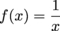.

Find any asymptotes by checking for which values make the denominator equal to zero.

In this case, f(x) is undefined for x = 0. This means that the line x = 0 (a.k.a. the y-axis) is a vertical asymptote.

Now for the intercepts:

We find the y-intercept by evaluating f(0). But for this case, f(0) is undefined. So there is no y-intercept.

We find the x-intercept by setting the numerator equal to zero. In this case, the numerator is simply 1. There is also no x-intercept.

However, the x-axis does act as a horizontal asymptote. The graph approaches the x-axis but will never touch it. Same goes for the y-axis.

Let's make a table to get a few more points.Now, plot our points.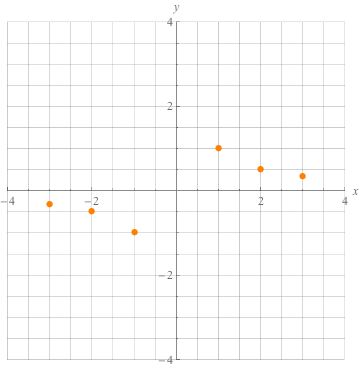Hmmmm, something tells us that we don't have enough information to finish the graph. Let's add a few more points to our table.Add those points and see what we get.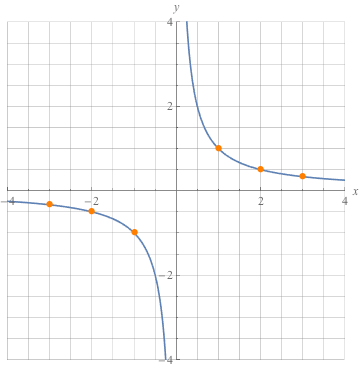### Sample Problem

Graph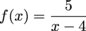.

Find any asymptotes by checking for x-values that turn the denominator into zero.

In this case, f(x) is undefined for x = 4. That tells us x = 4 is an asymptote. Let's sketch a dotted vertical line right there.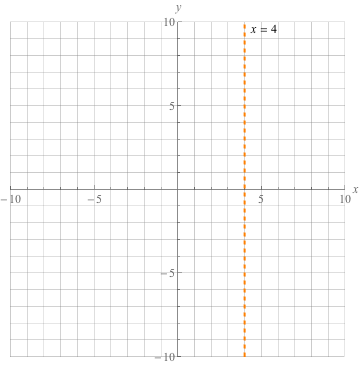Now for the intercepts. We find the y-intercept by evaluating f(0).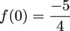So the y-intercept is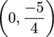.

We find the x-intercept by setting the numerator equal to zero. But in this case, the numerator is simply 5. So there is no x-intercept.

But once again, the x-axis acts as a horizontal asymptote. The graph will approach it but never cross it.

Let's make a table to get a few more points.Now we can plot the points from the table, with the asymptote at the undefined spot.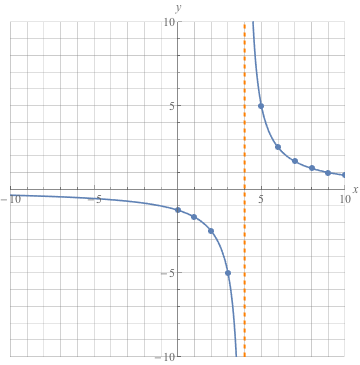### Sample Problem

Graph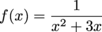.

Asymptote time. In this case, f(x) is undefined when the denominator is zero, which means:

x2 + 3x = 0

Factor and solve to find the zeros.

x(x + 3) = 0

So x = 0 and x + 3 = 0, giving us zeros of x = 0 and x = -3. Whoa, that means we have two vertical asymptotes at x = 0 and x = -3.

Sketch the axes, and draw in a dotted vertical line at x = -3. Don't forget, we've also got a vertical asymptote at x = 0, so imagine a dotted line over the y-axis too.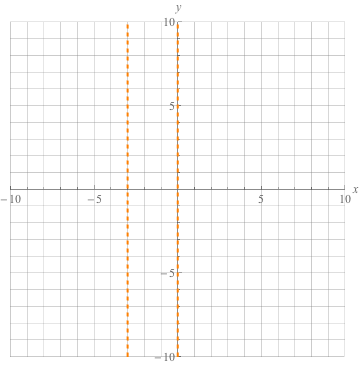Since x = 0 is an asymptote, we have no y-intercept.

And once again, we can't set the numerator equal to zero, so there's no x-intercept; however, the x-axis does act as a horizontal asymptote.

We need a table to get a few points.Plot the points from the table. And sketch in a graph using the x-axis, x = 0, and x = -3 as asymptotes.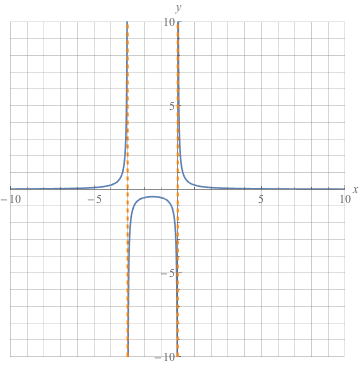• ### How to Solve Rational Equations

But wait, there's more. Solutions. How do we solve these bad boys?

A rational equation is an equation with at least one rational expression all up in it. That means fractions. To solve a rational equation for x (or whatever variable), we need to get rid of those beastly fractions. Here's how.

• Find the LCM of all the denominators.
• Multiply each term of the equation by the LCM.
• Solve just like you would with a linear or quadratic equation.

Not too bad. (For a refresher on what linear and quadratic equations are, check here and here.)

Any solutions that cause a denominator to be zero in the original equation are dirty, dirty liars. They're not really solutions at all. They should not pass "Go," nor should they collect \$200. Anytime we multiply by a variable or square a variable, we've got to check our solutions. Probably the easiest way to do this is by checking to see if our solutions are within our domain—which brings us to our next topic.

• ### Finding the Domain of Rational Functions

The domain of a rational function is all of the x-values that don't break the function. If a value of x doesn't make the denominator zero, it's part of the domain. If a value of x makes the function blow up, it's not part of the domain. These x-values become asymptotes. Sure, the first rule of rational functions is "we don't talk about rational functions," but the second rule is to never divide by zero. Don't do it. The rule breakers get kicked out of the domain. Ka-pow.

### Sample Problem

What's the domain of the following function?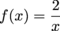The domain is everywhere the denominator isn't zero.

Here, the denominator will only be zero when x = 0.

So, our domain is -∞ < x < ∞, except x = 0. We can also write that as x ≠ 0.

### Sample Problem

What's the domain of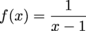?

For the domain, everything that doesn't make the denominator zero is fair game. This time, plugging in x = 1 will give us an undefined fraction, so our domain is -∞ < x < ∞, except x = 1. In other words, x ≠ 1.

### Sample Problem

What's the domain of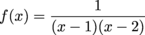?

Same old, same old. The domain is everywhere the denominator isn't zero. We'll get a zero in our denominator when x = 1 or x = 2. That tells us we've got a domain of -∞ < x < ∞, except x = 1 and x = 2. Or, to keep it short and sweet, the domain is x ≠ 1 and x ≠ 2.

• ### Solving Rational Equations

Now that we know domains and all that jazz, we can solve those pesky rational equations. Not a problem.

Here's the deal.

First, we'll find the LCM of all the denominators. Next, we'll multiply each term of the equation by the LCM. Then we'll solve just like we would with a linear or quadratic equation. No one said this would be excessively easy, but it's doable. Trust us.

One tricky thing to remember: Always be on the lookout for solutions that make the denominator zero. We'll call them extraneous solutions, and they don't count as actual solutions to the equation. They're mathematical Death Stars, destroying everything in their path. Always double check to make sure that this isn't the case.

### Sample Problem

Solve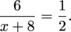We need to clear the equation of fractions.

The LCM of the denominators is 2(x +8). Multiply each term by the LCM like this: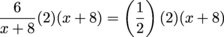Cancel identical factors.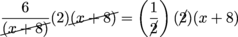6(2) = x + 8

12 = x + 8

Subtract 8 from both sides.

x = 4

Don't forget to check to make sure the solution doesn't blow up the denominator and that it works out properly. Double check using mental math, pen or pencil, or even abacus, but always check.

So does 4 check?

Does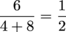?

Yes,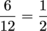.

An easier way to check would be to see if x = 4 is in the domain. The domain is everywhere but where the denominator is zero. In this example, that would be everywhere except x = -8.

So x = 4 is definitely in the domain, too. Check, double check.

### Sample Problem

Solve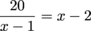.

First, multiply both sides by the LCM of the denominators. This time around, that's just (x – 1).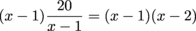Cancel those (x – 1) terms on the left.

20 = (x – 1)(x –2)

Multiply the right side out.

20 = x2 – 3x + 2

Subtract 20 from both sides.

x2 – 3x – 18 = 0

Factor the left side.

(x – 6)(x + 3) = 0

Set each factor equal to zero.

x – 6 = 0 and x + 3 = 0

Which give us solutions of: x = 6 and x = -3.

Now, see if they're extraneous solutions by checking if they're living in the domain.

The domain for this equation is everywhere where all denominators are not zero. The only denominator we need to deal with is x – 1.

So, the domain is everywhere except x = 1.

Both x = 6 and x = -3 are legit solutions.

Q.E.D. and bravo.

### Sample Problem

Solve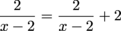.

First, multiply through by (x – 2).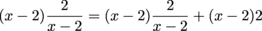Now cancel.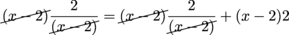2 = 2 + 2(x – 2)

2 = 2 + 2x – 4

2 = 2x – 2

2x = 4

x = 2

Now, check if this is an extraneous root. Does this cause a denominator in the original equation to be zero?

Yes, so x = 2 is an extraneous solution.